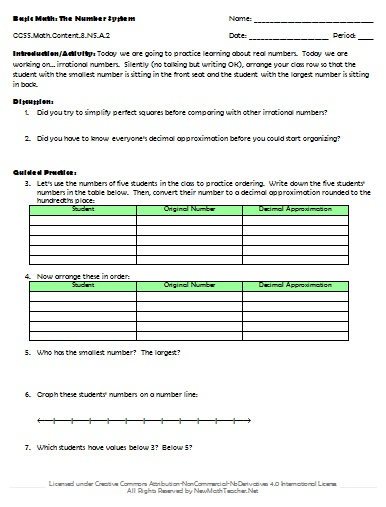# Engaging Math Lessons.

Please browse our selection of mathematics lessons sorted by subject and current common core standards for improving math achievement for every student.

## Basic Math: Grade 8

Below are a set of lesson plans and worksheets for Grade 8 Math that correspond to Common Core concepts and help students solve real-world problems. Lesson previews provide a quick glimpse at the provided materials.

• ### Concept:

The Number System
• Common Core Standard:CCSS.Math.Content.8.NS.A.1
• Description: Know that numbers that are not rational are called irrational. Understand informally that every number has a decimal expansion; for rational numbers show that the decimal expansion repeats eventually, and convert a decimal expansion which repeats eventually into a rational number.• ### Concept:

The Number System
• Common Core Standard:CCSS.Math.Content.8.NS.A.2
• Description: Use rational approximations of irrational numbers to compare the size of irrational numbers, locate them approximately on a number line diagram, and estimate the value of expressions. For example, by truncating the decimal expansion of √2, show that √2 is between 1 and 2, then between 1.4 and 1.5, and explain how to continue on to get better approximations.